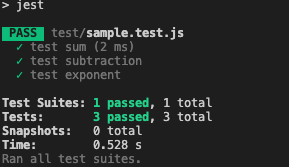### Introduction

This blog shows how to get start quickly to test with JavaScript.

### Init NPM repo and setup packages

Here we will setup the npm repo, then later install the dependencies package `jest`.

``````npm init -y
``````
``````npm install --save-dev jest
``````

### Add test Script at `package.json`

Add `test` command with `jest`, now if we run `npm run test`, should run jest with default setting.

``````  "scripts": {
"test": "jest"
},
``````

Here, create a file and create few JavaScript functions.

``````function sum(a, b) {
return a + b;
}

function exponent(a,b) {
return a ** b;
}

function subtraction(a, b) {
return a -b;
}
module.exports = {
sum,
subtraction,
exponent
};
``````

Next, create test file and add test code.

``````const {exponent, subtraction, sum} = require('../src/sample');

test('test sum', () => {
expect(sum(1, 2)).toBe(3);
});

test('test subtraction', () => {
expect(subtraction(10, 2)).toBe(8);
});

test('test exponent', () => {
expect(exponent(5, 2)).toBe(25);
});
``````

### Run the `npm run test`

That's it, now we can see the test passed.